# Multi-spectral Imaging¶

Important: Please read the installation page for details about how to install the toolboxes. $\newcommand{\dotp}{\langle #1, #2 \rangle}$ $\newcommand{\enscond}{\lbrace #1, #2 \rbrace}$ $\newcommand{\pd}{ \frac{ \partial #1}{\partial #2} }$ $\newcommand{\umin}{\underset{#1}{\min}\;}$ $\newcommand{\umax}{\underset{#1}{\max}\;}$ $\newcommand{\umin}{\underset{#1}{\min}\;}$ $\newcommand{\uargmin}{\underset{#1}{argmin}\;}$ $\newcommand{\norm}{\|#1\|}$ $\newcommand{\abs}{\left|#1\right|}$ $\newcommand{\choice}{ \left\{ \begin{array}{l} #1 \end{array} \right. }$ $\newcommand{\pa}{\left(#1\right)}$ $\newcommand{\diag}{{diag}\left( #1 \right)}$ $\newcommand{\qandq}{\quad\text{and}\quad}$ $\newcommand{\qwhereq}{\quad\text{where}\quad}$ $\newcommand{\qifq}{ \quad \text{if} \quad }$ $\newcommand{\qarrq}{ \quad \Longrightarrow \quad }$ $\newcommand{\ZZ}{\mathbb{Z}}$ $\newcommand{\CC}{\mathbb{C}}$ $\newcommand{\RR}{\mathbb{R}}$ $\newcommand{\EE}{\mathbb{E}}$ $\newcommand{\Zz}{\mathcal{Z}}$ $\newcommand{\Ww}{\mathcal{W}}$ $\newcommand{\Vv}{\mathcal{V}}$ $\newcommand{\Nn}{\mathcal{N}}$ $\newcommand{\NN}{\mathcal{N}}$ $\newcommand{\Hh}{\mathcal{H}}$ $\newcommand{\Bb}{\mathcal{B}}$ $\newcommand{\Ee}{\mathcal{E}}$ $\newcommand{\Cc}{\mathcal{C}}$ $\newcommand{\Gg}{\mathcal{G}}$ $\newcommand{\Ss}{\mathcal{S}}$ $\newcommand{\Pp}{\mathcal{P}}$ $\newcommand{\Ff}{\mathcal{F}}$ $\newcommand{\Xx}{\mathcal{X}}$ $\newcommand{\Mm}{\mathcal{M}}$ $\newcommand{\Ii}{\mathcal{I}}$ $\newcommand{\Dd}{\mathcal{D}}$ $\newcommand{\Ll}{\mathcal{L}}$ $\newcommand{\Tt}{\mathcal{T}}$ $\newcommand{\si}{\sigma}$ $\newcommand{\al}{\alpha}$ $\newcommand{\la}{\lambda}$ $\newcommand{\ga}{\gamma}$ $\newcommand{\Ga}{\Gamma}$ $\newcommand{\La}{\Lambda}$ $\newcommand{\si}{\sigma}$ $\newcommand{\Si}{\Sigma}$ $\newcommand{\be}{\beta}$ $\newcommand{\de}{\delta}$ $\newcommand{\De}{\Delta}$ $\newcommand{\phi}{\varphi}$ $\newcommand{\th}{\theta}$ $\newcommand{\om}{\omega}$ $\newcommand{\Om}{\Omega}$

This numerical tour explores multi-spectral image processing.

In :
addpath('toolbox_signal')


The multispectral image used in this tour is taken from the database of <http://www2.cmp.uea.ac.uk/Research/compvis/MultiSpectralDB.htm Hordley, Finlayson, Morovic> You can test the methods developped in this tour on other images.

Scilab user should increase memory. WARNING: This should be done only once.

In :
extend_stack_size(10);


## Multi-spectral Images¶

A multi-spectral image is a |(n,p,q)| cube of data, where |(n,p)| is the size of the image, and |q| is the number of spectral samples, ranging from infra-red to ultra-violet. The RGB channels are located approximately at samples locations |[10 15 20]|

In :
name = 'unclebens';
options.nbdims = 3;
n = 256;
M = rescale( crop(M,[n n size(M,3)]) );


width of the image

In :
n = size(M,1);


number of spectral components

In :
p = size(M,3);


Display a few channels of the image.

In :
clf;
imageplot(M(:,:,10), 'R', 1,3,1);
imageplot(M(:,:,15), 'G', 1,3,2);
imageplot(M(:,:,20), 'B', 1,3,3);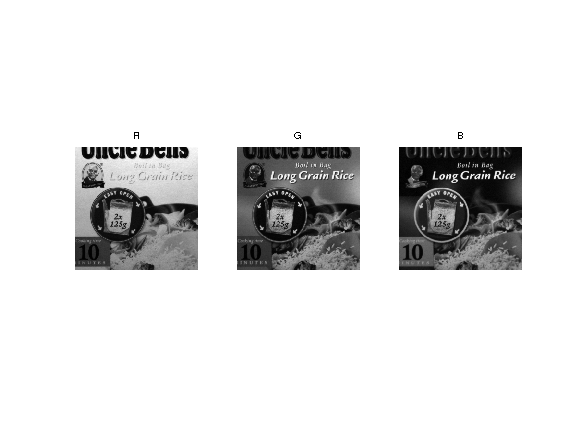Display an approximate RGB image.

In :
rgbsel = [10 15 20];
clf;
imageplot(M(:,:,rgbsel), 'RGB');Display the spectral content of a given pixel. As you can see, spectral curves are quite smooth.

pixel location

In :
pos = [30 50];


spectral content

In :
v = M(pos(1),pos(2),:); v = v(:);
clf;
plot( v, '.-');
axis('tight');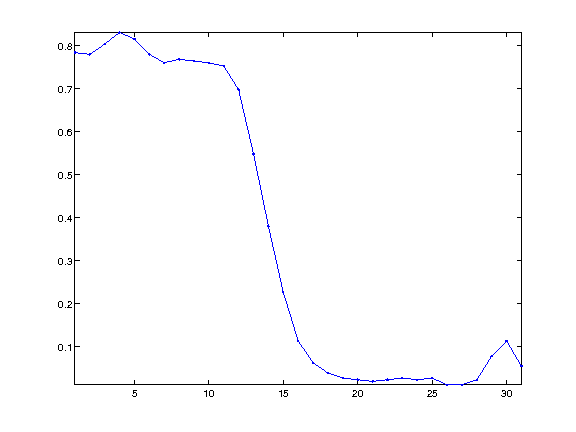## Multi-Spectral Image Compression¶

To perform the compression / approximation of the full cube of data, one needs to use a 3D transformation of the cube. One can use a truely 3D wavelet transform, or the combination (tensor product) of a 2D wavelet transform and a cosine transform.

A 1D DCT is first applied to each spectral content.

In :
U = reshape( M, [n*n p] )';
U = dct(U);
U = reshape(U', [n n p]);


We plot the spectral content of a pixel and its DCT transform. You can note that the DCT coefficients are quikcly decaying.

In :
clf;
subplot(2,1,1);
v = M(pos(1),pos(2),:); v = v(:);
plot(v); axis('tight');
title('Spetral content');
subplot(2,1,2);
v = U(pos(1),pos(2),:); v = v(:);
plot(v); axis('tight');
title('DCT tranform');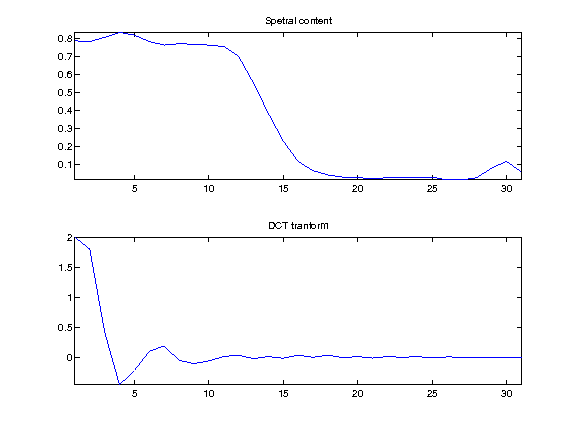As the frequenc index |i| increase, the DCT component |U(:,:,i)| becomes small and noisy. Note that |U(:,:,1)| is the average of the spectral components.

In :
ilist = [1 4 8 16];
clf;
for i=1:length(ilist);
imageplot(U(:,:,ilist(i)), ['DCT freq ' num2str(ilist(i))], 2,2,i);
end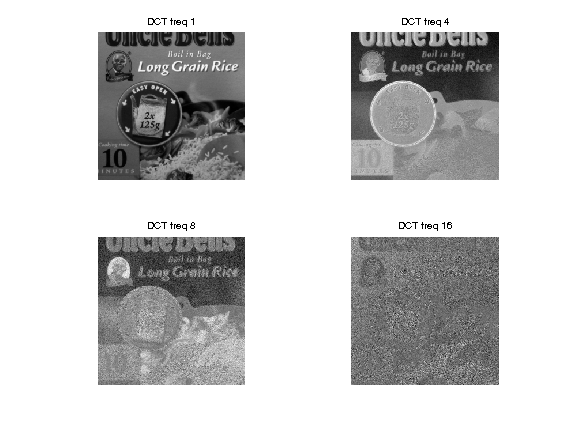The tensor product transform is obtained by applying a 2D transform.

In :
Jmin = 3;
UW = U;
for i=1:p
UW(:,:,i) = perform_wavelet_transf(U(:,:,i), Jmin, +1);
end


Display two differents sets of wavelets coefficients

In :
clf;
subplot(1,2,1);
plot_wavelet(UW(:,:,1), Jmin);
subplot(1,2,2);
plot_wavelet(UW(:,:,10), Jmin);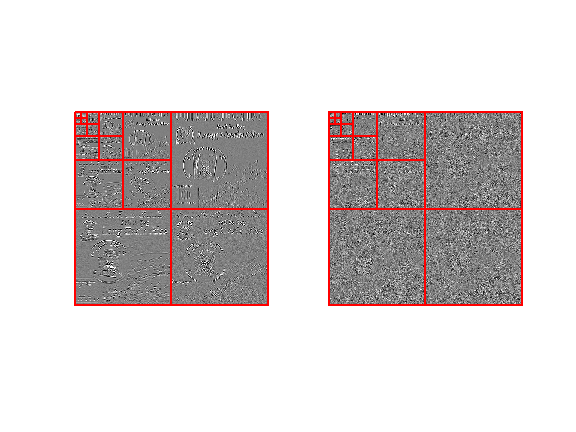Approximation is obtained by thresholding the coefficients.

number of kept coefficients

In :
m = round(.01*n*n*p);


threshold to keep only m coefficients

In :
UWT = perform_thresholding(UW, m, 'largest');


Exercise 1

Implement the inverse transform to recover an approximation |M1| from the coefficients |UWT|. proximation error isplay

In :
exo1()In :
%% Insert your code here.


Exercise 2

Compare the approximation error (both in term of SNR and visually) of a multispectral image with a 3D Haar basis and with a tensor product of a 2D Haar and a DCT.

In :
exo2()

In :
%% Insert your code here.


## Multi-Spectral Image Denoising¶

A redundant representation of the multi-spectral image is obtained by using a DCT along the spectral dimension (3rd dimension) and a 2D translation invariant wavelet transform of the spacial dimension.

Exercise 3

Compare the denoising (both in term of SNR and visually) of a multispectral image with an independant thresholding of each channel within a translation invariant 2D wavelet basis, and with a thresholding of the DCT/invariant wavelet representation. For each method, compute the optimal threshold value.

In :
exo3()

In :
%% Insert your code here.